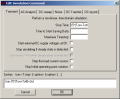# How to calculate LED average power???

#### RATPL

Joined May 7, 2015
1
Hi everyone,

I am using LED Vf=3v and If=700mA. This LEDs used some light array project as flashing leds simultanously.

i will turn on 15 LEDs at a time. 5x3 (5rows and 3 coloum) matrix.

Duty cycle is 667ms

ON time=165mS
OFF time=502mS

LES are 2W rated .

#### StayatHomeElectronics

Joined Sep 25, 2008
1,070
Instantaneous power of the individual LED is equal to the voltage across the LED multiplied by the current through the LED. When the signal is being turned on and off you can multiply the power by the duty cycle to get the average power. The duty cycle is the time the device is on divided by the complete cycle time.

#### Alec_t

Joined Sep 17, 2013
12,248
If the LEDs are on for 165mS then you also need to know the instantaneous power they consume, to ensure you're not overloading the power supply. Things can get pretty hot in 165mS !

#### ErnieM

Joined Apr 24, 2011
8,183
Ok, so you have 15 LEDs each drawing .7 A so that is .7x15=10.5 amps instantaneous current. Your supply should be rated for at least that, and more is better.

The instantaneous power is just V x I or 3V x 10.5A = 31.5 watts.

To account for the duty cycle you just need to make a ratio of on time to total time, or 165 / 667 which is just about 25 percent. So the average power is just .25 x 31.5 = 7.8 watts.

#### Bordodynov

Joined May 20, 2015
2,916
One LED Power=Vf*If*(ON time)/(Duty cycle)=3V*0.7A*(165/677)=0.512W=512mW
15 Led Power15=0.512W*15=7.68W

#### spinnaker

Joined Oct 29, 2009
7,835
Or let LTSpice do it for you...

View attachment 93222

I doubt that V(f) = 3.000V
Not to hijack the OP's thread but could you show how this was done?

I tried and it is running forever for me. If you can show me what I did wrong then I will add a little tutorial on how to do it. It might help us LTSpice newbies.

I added a current source with the following values.